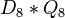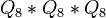# Extraspecial group

The article defines a property of groups, where the definition may be in terms of a particular prime that serves as parameter
View other prime-parametrized group properties | View other group properties

## Definition

A group of prime power order (or, more generally, any p-group) is termed extraspecial if its center, derived subgroup and Frattini subgroup all coincide, and moreover, each of these is a group of prime order (and hence, a cyclic group).

## Classification and particular cases

Any finite extraspecial group has order$p^{2r + 1}$ for some positive integer$r$. For each$r$, there are two extraspecial groups (up to isomorphism), denoted the + and - types respectively.

### The case$p = 2$

All the extraspecial groups are obtained as iterated central products of copies of dihedral group:D8 and quaternion group. The$+$ type corresponds to the cases where the quaternion group part occurs an even number of times in the central product and the$-$ type corresponds to the case where the quaternion group occurs an odd number of times.$r$ Order$2^{2r + 1}$ Group of$+$ type Central product expressions GAP ID (second part) Group of$-$ type Central product expressions GAP ID (second part)
1 8 dihedral group:D8$D_8$ 3 quaternion group$Q_8$ 4
2 32 inner holomorph of D8$D_8 * D_8$,$Q_8 * Q_8$ 49 central product of D8 and Q8$D_8 * Q_8$ 50
3 128  ?$D_8 * D_8 * D_8$,$Q_8 * Q_8 * D_8$ 2326  ?$D_8 * D_8 * Q_8$,$Q_8 * Q_8 * Q_8$ 2327